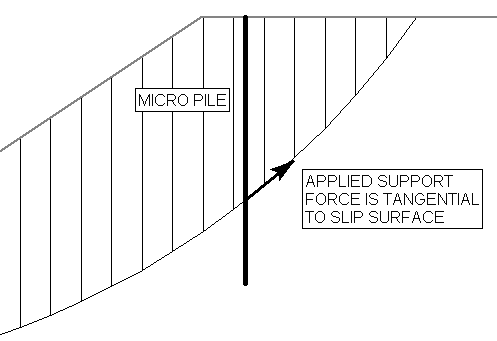Join one of our upcoming courses taking place around the world! Explore now

# Pile/Micropile

The Pile/Micro Pile support type can be used to model a micro pile or pile type of support system. Due to the nature of pile support, this type of support behaves differently from other types of support in Slide3, since resistance to movement is assumed to be provided by loading transverse to the support direction, rather than parallel to the support direction. Note:

• Like all other support types in Slide3, it is necessary for a slip surface to intersect a Micro Pile, in order for the support to have an effect on the safety factor of the slip surface.
• However, unlike other support types in Slide3, the only failure mode for a Micro Pile, is by Shear, transversely through the pile (this is illustrated below).
• Tension or pullout are NOT considered as failure modes for a Micro Pile in Slide3, regardless of the pile orientation. Only transverse shear through the pile is considered.
Make sure that you have read the Overview of Support Implementation topic, for important information which applies to all support models in Slide3.

## Pile Shear Strength

The Pile Shear Strength, is the shear FORCE necessary to cause shear failure THROUGH the pile. For illustration, the diagram below is 2D however the actual implementation is 3-dimensional.

The Pile Shear Strength is entered as a FORCE. The user must calculate the total shear force capacity of a pile, based on the pile cross-sectional dimensions, and the pile shear strength per unit area of cross-section.

## Force Application

By default, if Support Type = Micro Pile, the method of Force Application = Active. See the Force Application topic for a discussion of the significance of Active and Passive support force application in Slide3.

## Force Orientation

When a slip surface intersects a Micro Pile, the default orientation of the applied force (i.e. the Pile Shear Strength) is Parallel to Surface. For a given column base, this means that the force will be applied in the plane of the column base, against the direction of sliding. This is illustrated below in 2D; the actual implementation is 3D. This force orientation is consistent with the assumed failure mode of a Micro Pile. You can also choose Perpendicular to Pile as the force orientation if you want the applied force to be exactly perpendicular to the pile regardless of the slip surface orientation.Applied force orientation for micro pile, parallel (tangential) to surface diagram

## Implementation of Pile Support in Slide3

As discussed above, a Pile will apply a constant force (magnitude) to a slip surface, regardless of where it is intersected by a slip surface. The applied load is simply equal to the Pile Shear Strength:

F = P

where:

P = Pile Shear Strength (force units)

The Force Diagram for a Pile support type, is therefore a horizontal line, representing a constant value of applied force, regardless of where a slip surface intersects the pile.